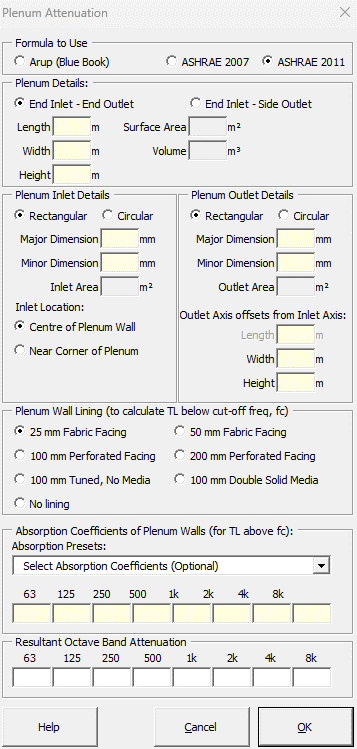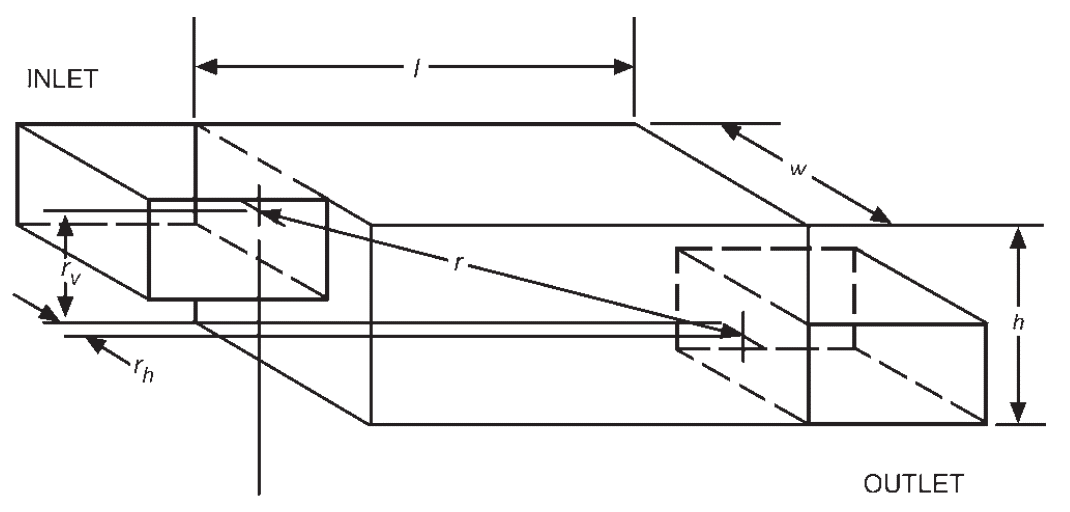### Strutt Help

Plenum Attenuation    1/1

Strutt|Mechanical Services|Duct Elements|Plenum inserts the octave band attenuation from a plenum into the active row of the worksheet.The Arup Mechanical Services Guide (Wells) method calculates attenuation from a plenum using:

Attenuation = -10log_10 (S_2 (cos(theta)/(2 pi d^2) + 1/R_c))

where,
S_o is the outlet area (m2)
d is the slant distance inlet/outlet (m)
theta is the angle d makes with the inlet axis (deg)
R_c is the Room Constant and is derived from:

R_c = (S_o + S_i + S bar(alpha))/(1 - bar(alpha))

where,
S is the total internal surface area of the plenum (m2) excluding the inlet and outlet area
S_i is the plenum inlet area (m˛)
bar(alpha) is the average absorption coefficient of the internal walls of the plenum excluding the openings
Strutt assumes that the inlet and outlet openings have alpha = 1 and calculates the overall room constant accordinglyInputs required in the calculation are:

• Length, width and height of plenum (not including inlet/outlet ducts), used to calculate total internal surface area of plenum.
• Inlet and Outlet dimensions, used to calculate total internal surface area of plenum.
• Outlet axis offsets from inlet axis, used to calculate slant distance and the angle of the slant distance with inlet axis.
• Absorption coefficients of the plenum lining (alternatively, coefficients for common lining materials may be selected from the drop-down menu.

Note: use both methods with caution where either the width or the height of the plenum is less than 1.5d, in which case the plenum may not act as an expansion chamber

ASHRAE 2007 calculates attenuation from a plenum as follows:
Plane wave propagation in a duct exists at frequencies below the cutoff, creating the need to consider two frequency ranges. The cutoff frequency f_(co) is the frequency above which plane waves no longer propagate in the duct.

f_(co) = c/(2a)

or

f_(co) = 0.586 c/d

where,
f_(co) is the cutoff frequency (Hz)
c is the speed of sound in air (m/s)
a is the larger cross-sectional dimension of rectangular duct (m)
d is the diameter of a round duct (m)

Table 7 ASHRAE gives sound absorption coefficients for common plenum materials. These are incorporated into the calculation and the appropriate material can be chosen.
At the frequencies above fco, waves that propagate in the duct are called cross or spinning modes. The TL in this higher frequency range is predicted using:

TL = b [(S_(out) Q)/(4 pi r^2) + (S_(out) (1 - alpha_a))/(S alpha_a)]^n

TL = b (K_f)^n

where,
TL is the transmission loss (dB)
b = 3.505
n = -0.359
K_f is the attenuation coefficient
S_(out) is the area of plenum outlet (m2)
S is the total inside surface area of plenum minus inlet and outlet areas (m2)
r is the distance between centers of inlet and outlet of plenum (m)
Q is the directivity factor; taken as 2 for opening near centre of wall, 4 for opening near corner of plenum
alpha_a is the average absorption coefficient of plenum lining given by

alpha_a = (S_1 alpha_1 + S_2 alpha_2)/(S_1 + S_2)

where,
alpha_1 is the sound absorption coefficient of any bare or unlined inside surfaces of plenum
S_1 is the surface area of any bare or unlined inside surfaces of plenum (m2)
alpha_2 is the sound absorption coefficient of acoustically lined inside surfaces of plenum
S_2 is the surface area of acoustically lined inside surfaces of plenum (m2)

For lower frequencies below fco (with a limit of 50 Hz), TL is calculated as:

TL = 10.76 * A_f S + W_e

where,
10.76 is a conversion factor from square metres to sqaure feet
A_f is the surface area coefficient (dB/ft2), referenced according to plenum size. NOTE: the coefficients in Table 13 in the SI version of the Handbook are incorrect - the coefficients from the IP Handbook are used instead, and the 10.76 correction factor is applied.
W_e is the wall effect (dB), selected by the user as the type of plenum wall lining

Other corrections that are taken into account in the calculation of TL at all frequencies are:

End-in/End-out configurations where the openings are not in-line, the influence of offset angle is included as a correction.

cos(theta) = l/r = l/sqrt(l^2 + r_w^2 + r_h^2)

where,
theta is the offset angle representring r to long axis l of duct
l is the length of plenum (m)
r_w is the vertical offset between axes of plenum inlet and outlet (m)
r_h is the horizontal offset between axes of plenum inlet and outlet (m)
Note that r_w and r_h are interchangeable.

For a given offset angle, Table 9 of the ASHRAE guide includes a TL correction for angles up to 45°.

End-in/Side-out configurations where openings are perpendicular to each other, the elbow effect is included as a correction using the values in Table 10 ASHRAE.

ASHRAE 2011 implements the same formula as ASHRAE 2007. However, ASHRAE 2011 recommends that the Transmission Loss below the cutoff frequency should be limited to 20 dB. It is recommended that ASHRAE 2011 be used instead of ASHRAE 2007 to avoid unrealistically large low frequency transmission loss values, which can occur in some circumstances.

References:

• Arup Sound and Vibration in Mechanical Services Design, p46
• ASHRAE 2007 Handbook, 47.12 - 47.15
• ASHRAE 2011 Handbook, 48.18 - 48.21